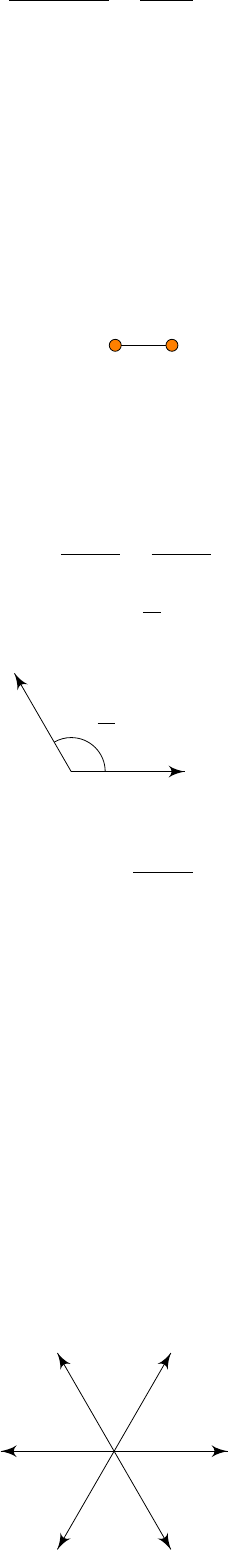5Cartan classification

III Symmetries, Fields and Particles5.7 Reconstruction
Now given the Cartan matrix, we want to reconstruct the Lie algebra itself.
Recall that we had a Cartan-Weyl basis
{H
i
, E
α
: i = 1, ··· , r : α Φ}.
The first thing to do in the reconstruction is to figure out what the set of roots
is.
By definition, the Cartan matrix determines simple roots
α
(i)
for
i
= 1
, ··· , r
.
We can read off the inner products from the Cartan matrix as
A
ij
=
2(α
(i)
, α
(j)
)
(α
(j)
, α
(j)
)
=
2|α
(i)
|
|α
(j)
|
cos ϕ
ij
.
This allows us to find the simple roots.
How about the other roots? We can find them by considering the root strings,
as we know that the length of the α
(i)
-string through α
(j)
is given by
`
ij
= 1 A
ji
N.
So we can work out the length of the root string from each simple root. By
Cartan’s theorem, this gives us all roots.
Instead of going through a formal and general reconstruction, we do an
example.
Example. Consider g = A
2
. We have a Dynkin diagram
A
2
:
So we see that the Cartan matrix is
A =
2 1
1 2
.
So we know that g has two simple roots α, β Φ, and we know
A
12
= A
21
=
2(α, β)
(α, α)
=
2(β, α)
(β, β)
= 1.
So we find that (α, α) = (β, β), and ϕ
αβ
=
2π
3
. So we can display the roots as
α
β
2π
3
Since α, β Φ
S
, we know ±(α β) 6∈ Φ, and also we have
`
α,β
= `
β
= 1
2(α, β)
(α, α)
= 2.
So we have roots
β
+
nα, α
+
˜
for
n, ˜n {
0
,
1
}
. So in fact our list of roots
contains
α, β, α + β Φ.
These are the positive ones. We know each of these roots has a negative
counterpart. So we have more roots
α, β, α β Φ.
Then by our theorem, we know these are all the roots. We can find the length
of α + β by
(α + β, α + β) = (α, α) + (β, β) + 2(α, β)
= (α, α) + (β, β) (β, β)
= (α, α).
So in fact all roots have the same length. So if we draw all our roots, we get
α
β
α + β
α
βα β
So the Cartan-Weyl basis consists of
B
CW
= {H
1
, H
2
, E
±α
, E
±β
, E
±(α+β)
}.
This is the complete Cartan-Weyl basis of
A
2
. So this has dimension 2 + 6 = 8.
If we want to find out the brackets of these things, we look at the ones we
have previously written down, and use the Jacobi identity many times.